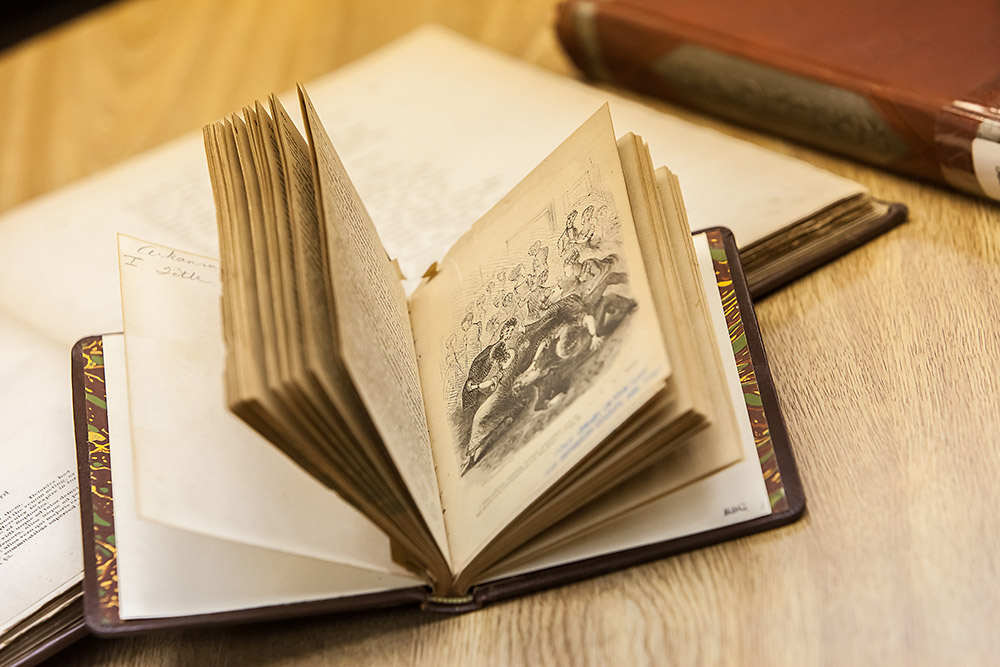Parents can register their children for the 2020 Summer Reading Program by going to the following link: http://pbjcls.readsquared.com/
The age groups are as follow:

Pre-K: Ages 0 - 4
Children: Ages 5 - 13
Teens: Ages 13 - 17

Points can be obtained for each book that is read. This can be accomplished by reading by minutes, days, pages, E-books, and Audiobooks.

Pre - K:
1 book = 30 points (for every book read that will be 30 points).
1 page = 5 points
1 paragraph = 1 point(s)
1 minute(s) = 1 point(s)
1 Day(s) = 35 points
1 Audiobooks = 25 points
1 E-book = 15 points
1 picture books = 30 points

Children
1 book(s) = 30 points
1 page(s) = 25 points
1 minute(s) = 1 point(s)
1 Day (s) = 30 points
1 Audiobook(s) = 25 points
1 E-book = 40 points

Teens
1 Book(s) = 60 points
1 Page(s) = 1 point
1 Minute(s) = 1 point(s)
1 Day(s) = 35 points
1 Audio Book(s) = 40 points
1 E-Book = 25 points

1 Book(s) = 25 points
1 Page(s) = 1 point(s)
1 Minute(s) = 1 point(s)
1 Day(s) = 20 points
1 Audiobook(s) = 15 points
1 E-Book(s) = 25.00

Participants can accumulate up to 1000 points per day. For the children, there are 9 badge levels:

100 pts. level 1
200 pts. level 2 up to level 9 which is the highest level to be completed. At the end of level 9, there will be a badge prize.

Be sure to register online at http://pbjcls.readsquared.com/  and join in the fun.

200 E. 8th Avenue
Pine Bluff, AR 71601
(870) 534-4802
Email Us